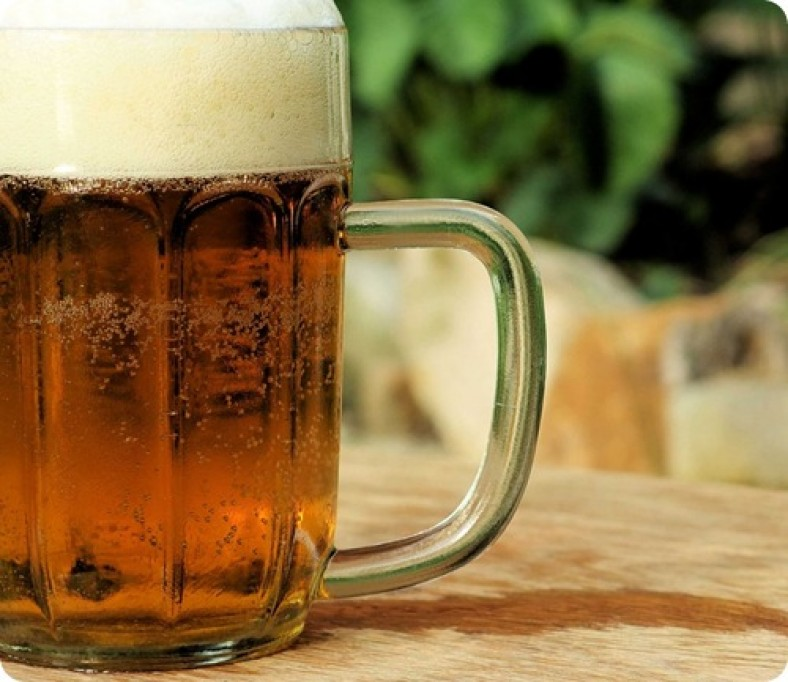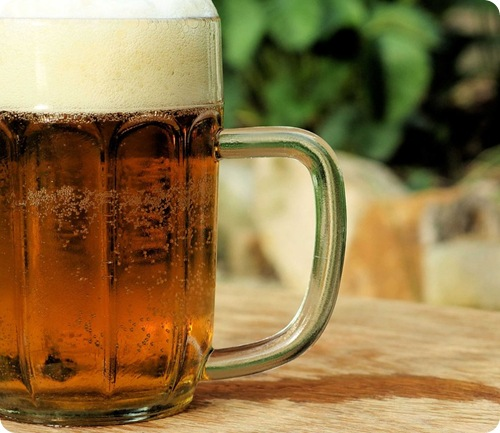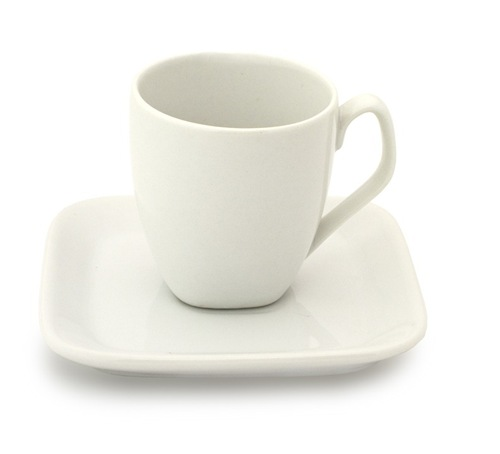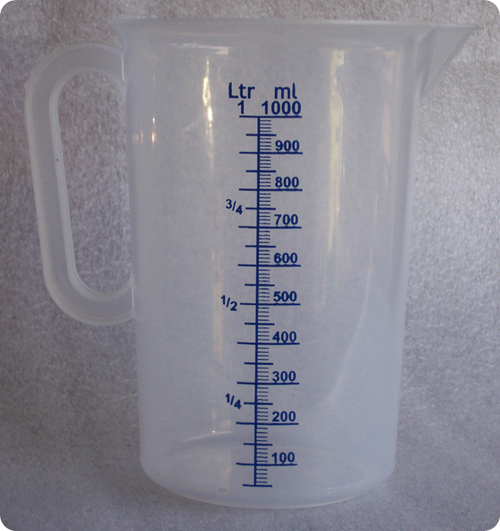# How many pints in a quart (cup, liter or pound)

How many pints in a quart? The pint is commonly used to measure liquids like water, beer and vinegar. It is written as “P”, “pt.” or “p”. It is the unit of capacity or volume in both the United States mathematical terms and British mathematical measurement systems. In the two of the system the pint is conventionally one-eighth (1 / 8) of a regular gallon. The British pint is about 20% bigger than the US pint as both the systems are not well-matched. Almost all other countries have been consistent on the metric system, so the size of what may be called a pint differs depending on native custom.

Related articles about How many pints in a quart

How many oz. in a cup (gallon, pound, liter, quart, pint, lb., shot glass, ml, gram)

How many liters in a gallon (quart, milliliter, cup, pint, ounce)

How many cups in a gallon (quart, pint, liter, pound, ounce, oz)

The British value for pint is 568 ml and is used in Ireland and UK and in Commonwealth nations. In the United States, there are 2 types of pints. One is a liquid pint and the other is a solid pint. A liquid pint is approximately equal to 473 ml and a less common one is the dry pint which is around 551 ml. Each of these pints is one-eighth of its particular. The difference in the gallons started from1824, when the British Weights and Measures Act standardized various liquid measures throughout the British Empire, while the United States continued to use the earlier English measures. The British pint is equal to 20 fluid ounces and the liquid pint of the US is 16 US fluid ounces.  Countries like as New Zealand, South Africa, Canada and Australia uses the pint but not the value they used in ancient times.Let us calculate how many pints make a quart.

1 pint is equal to 0.125 gallons.  0.125 gallons is equal to 0.5 quart. Therefore, we get 1 pint is a half quart.

In mathematical terms,

1 pint = 0.125 gallons AND 0.125 gallons = 0.5 quart

1 pint = 0.5 quart

2 pints = 0.5 X 2 = 1 quart

2 pints in a quart

How many pints in a cup0.5 pints are in a cup.

We know that 8 ounces make a cup. We know that 1 gallon is equal to 128 ounce.  Therefore, a cup has

0.06340132813 gallon. 0.5 pints are in 0.0625 gallon.

0.06340132813 is roughly equal to 0.0625 gallon.

0.5 pints is equal to 8.1 ounce (1 cup).

1 cup has 0.5 pints.

How many pints in a liter2.1 pints in a liter

1 pint is equal to 0.473176 liter. Let us calculate how many pints are in a liter.

0.473176 liter is equal to 1 pint. 1 pint = 0.473176 liter

0.473176 l = 1 pint

1 li = (1 / 0.473176) = 2.113378531455526

We can write it as 2.1 pints.

How many pints in a pound1 pint in a pound

A pint is made of 2 cups.  One cup is equal to 8 ounces.  Therefore, 2 cups are equal to 16 oz.  16 ounces is equal to 1 pound. Therefore, we can conclude that there is 1 pint in a pound.

1 pint = 2 cups, 2 cups = 16 oz.

16 oz. = 1 lb.

We can equate them and say 1 pint = 1 lb.

This site uses Akismet to reduce spam. Learn how your comment data is processed.